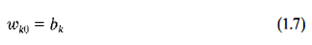Introduction:-A neuron is an information-processing unit that is fundamental to the operation of a neural network. The model of a neuron, which forms the basis for designing neural networks. Here we identify three basic elements of the neuronal model:-

1. A set of synapses or connecting links, each of which is characterized by a weight or strength of its own. Specifically, a signal Xj a t the input of synapse j connected to neuron k is multiplied by the synaptic weight wkj" It is important to make a note of the manner in which the subscripts of the synap tic weight wkj are written. The first subscript refers to the neuron in ques tion and the second subscrip t refers to the input end of the synapse to which the weight refers. Unlike a synapse in the brain, the synaptic weight of an artificial neuron may lie in a range that includes negative as well as positive values.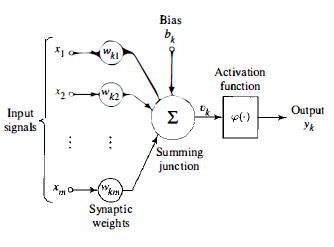2.An adder for summing the input signals, weighted by the respective synapses of the neuron; the operations described here constitute a linear combiner.

3. An activation function for limiting the amplitude of the output of a neuron. The activation function is also referred to as a squashing function in that it squashes the permissible amplitude range of the output signal to some finite value.

Typically, the normalized amplitude range of the output of a neuron is written as the closed unit interval [0, 1] or alternatively [- 1, 1]. The neuronal model also includes an externally applied bias, denoted by bk.The bias bk has the effect of increasing or lowering the net input of the activation function,depending on whether it is positive or negative, respectively.In mathematical terms, we may describe a neuron k by writing the following pair of equations: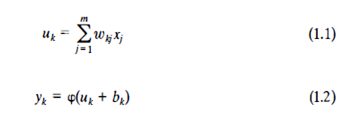Where the input signals are\are the synaptic weights of neuron k; Ukis the linear combiner output due to the input signals; bkis the bias;is the activation function; and Ykis the output signal of the neuron. The use of bias bkhas the effect of applying an affine transformation to the output Ukof the linear combiner depends on whether the bias bk is positive or negative, the relationshipbetween the induced local field or activation potential Vk of neuron k and the linear combiner output Uk is modified; hereafter the term "induced local field" is used. Note that as a result of this affine transformation, the graph of Vk versus Uk no longer passes through the origin.

The bias bk is an external parameter of artificial neuron k. We may account for its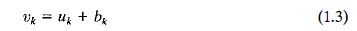In particular, depending on whether the bias bk is positive or negative, the relationship between the induced local field or activation potential Vkof neuron k and the linear combiner output Ukis modified in the manner; hereafter the term "induced local field" is used. Note that as a result of this affine transformation, the graph of Vkversus Ukno longer passes through the origin. The bias bkis an external parameter of artificial neuron k. We may account for its presence as in Eq. (1.2). Equivalently, we may formulate the combination of Eqs. (1.1)to (1.3) as follows: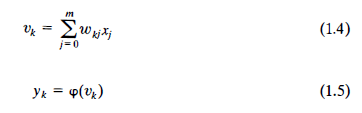In Eq. (1.4) we have added a new synapse. Its input is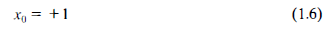And its weight is,